# 6.3: Paired-samples t-test

$$\newcommand{\vecs}{\overset { \rightharpoonup} {\mathbf{#1}} }$$ $$\newcommand{\vecd}{\overset{-\!-\!\rightharpoonup}{\vphantom{a}\smash {#1}}}$$$$\newcommand{\id}{\mathrm{id}}$$ $$\newcommand{\Span}{\mathrm{span}}$$ $$\newcommand{\kernel}{\mathrm{null}\,}$$ $$\newcommand{\range}{\mathrm{range}\,}$$ $$\newcommand{\RealPart}{\mathrm{Re}}$$ $$\newcommand{\ImaginaryPart}{\mathrm{Im}}$$ $$\newcommand{\Argument}{\mathrm{Arg}}$$ $$\newcommand{\norm}{\| #1 \|}$$ $$\newcommand{\inner}{\langle #1, #2 \rangle}$$ $$\newcommand{\Span}{\mathrm{span}}$$ $$\newcommand{\id}{\mathrm{id}}$$ $$\newcommand{\Span}{\mathrm{span}}$$ $$\newcommand{\kernel}{\mathrm{null}\,}$$ $$\newcommand{\range}{\mathrm{range}\,}$$ $$\newcommand{\RealPart}{\mathrm{Re}}$$ $$\newcommand{\ImaginaryPart}{\mathrm{Im}}$$ $$\newcommand{\Argument}{\mathrm{Arg}}$$ $$\newcommand{\norm}{\| #1 \|}$$ $$\newcommand{\inner}{\langle #1, #2 \rangle}$$ $$\newcommand{\Span}{\mathrm{span}}$$$$\newcommand{\AA}{\unicode[.8,0]{x212B}}$$

For me (Crump), many analyses often boil down to a paired samples t-test. It just happens that many things I do reduce down to a test like this. I am a cognitive psychologist, I conduct research about how people do things like remember, pay attention, and learn skills. There are lots of Psychologists like me, who do very similar things.

We all often conduct the same kinds of experiments. They go like this, and they are called repeated measures designs. They are called repeated measures designs, because we measure how one person does something more than once, we repeat the measure. So, I might measure somebody doing something in condition A, and measure the same person doing something in Condition B, and then I see that same person does different things in the two conditions. I repeatedly measure the same person in both conditions. I am interested in whether the experimental manipulation changes something about how people perform the task in question.

## Mehr, Song, and Spelke (2016)

We will introduce the paired-samples t-test with an example using real data, from a real study. Mehr, Song, and Spelke (2016) were interested in whether singing songs to infants helps infants become more sensitive to social cues. For example, infants might need to learn to direct their attention toward people as a part of learning how to interact socially with people. Perhaps singing songs to infants aids this process of directing attention. When an infant hears a familiar song, they might start to pay more attention to the person singing that song, even after they are done singing the song. The person who sang the song might become more socially important to the infant. You will learn more about this study in the lab for this week. This example, prepares you for the lab activities. Here is a brief summary of what they did.

First, parents were trained to sing a song to their infants. After many days of singing this song to the infants, a parent came into the lab with their infant. In the first session, parents sat with their infants on their knees, so the infant could watch two video presentations. There were two videos. Each video involved two unfamiliar new people the infant had never seen before. Each new person in the video (the singers) sang one song to the infant. One singer sang the “familiar” song the infant had learned from their parents. The other singer sang an “unfamiliar” song the infant had not hear before.

There were two really important measurement phases: the baseline phase, and the test phase.

The baseline phase occurred before the infants saw and heard each singer sing a song. During the baseline phase, the infants watched a video of both singers at the same time. The researchers recorded the proportion of time that the infant looked at each singer. The baseline phase was conducted to determine whether infants had a preference to look at either person (who would later sing them a song).

The test phase occurred after infants saw and heard each song, sung by each singer. During the test phase, each infant had an opportunity to watch silent videos of both singers. The researchers measured the proportion of time the infants spent looking at each person. The question of interest, was whether the infants would spend a greater proportion of time looking at the singer who sang the familiar song, compared to the singer who sang the unfamiliar song.

There is more than one way to describe the design of this study. We will describe it like this. It was a repeated measures design, with one independent (manipulation) variable called Viewing phase: Baseline versus Test. There was one dependent variable (the measurement), which was proportion looking time (to singer who sung familiar song). This was a repeated measures design because the researchers measured proportion looking time twice (they repeated the measure), once during baseline (before infants heard each singer sing a song), and again during test (after infants head each singer sing a song).

The important question was whether infants would change their looking time, and look more at the singer who sang the familiar song during the test phase, than they did during the baseline phase. This is a question about a change within individual infants. In general, the possible outcomes for the study are:

1. No change: The difference between looking time toward the singer of the familiar song during baseline and test is zero, no difference.
2. Positive change: Infants will look longer toward the singer of the familiar song during the test phase (after they saw and heard the singers), compared to the baseline phase (before they saw and heard the singers). This is a positive difference if we use the formula: Test Phase Looking time - Baseline phase looking time (to familiar song singer).
3. Negative change: Infants will look longer toward the singer of the unfamiliar song during the test phase (after they saw and heard the singers), compared to the baseline phase (before they saw and heard the singers). This is a negative difference if we use the same formula: Test Phase Looking time - Baseline phase looking time (to familiar song singer).

## The Data

Let’s take a look at the data for the first 5 infants in the study. This will help us better understand some properties of the data before we analyze it. We will see that the data is structured in a particular way that we can take advantage of with a paired samples t-test. Note, we look at the first 5 infants to show how the computations work. The results of the paired-samples t-test change when we use all of the data from the study.

Here is a table of the data:

library(data.table)
suppressPackageStartupMessages(library(dplyr))
"https://stats.libretexts.org/@api/deki/files/10603/MehrSongSpelke2016.csv")
experiment_one <- all_data %>% filter(exp1==1)
paired_sample_df <-  data.frame(infant=1:5,
Baseline = round(experiment_one$Baseline_Proportion_Gaze_to_Singer[1:5], digits=2), Test = round(experiment_one$Test_Proportion_Gaze_to_Singer[1:5],
digits=2))
knitr::kable(paired_sample_df)
infant Baseline Test
1 0.44 0.60
2 0.41 0.68
3 0.75 0.72
4 0.44 0.28
5 0.47 0.50

The table shows proportion looking times toward the singer of the familiar song during the Baseline and Test phases. Notice there are five different infants, (1 to 5). Each infant is measured twice, once during the Baseline phase, and once during the Test phase. To repeat from before, this is a repeated-measures design, because the infants are measured repeatedly (twice in this case). Or, this kind of design is also called a paired-samples design. Why? because each participant comes with a pair of samples (two samples), one for each level of the design.

Great, so what are we really interested in here? We want to know if the mean looking time toward the singer of the familiar song for the Test phase is higher than the Baseline phase. We are comparing the two sample means against each other and looking for a difference. We already know that differences could be obtained by chance alone, simply because we took two sets of samples, and we know that samples can be different. So, we are interested in knowing whether chance was likely or unlikely to have produced any difference we might observe.

In other words, we are interested in looking at the difference scores between the baseline and test phase for each infant. The question here is, for each infant, did their proportion looking time to the singer of the familiar song, increase during the test phase as compared to the baseline phase.

## The difference scores

Let’s add the difference scores to the table of data so it is easier to see what we are talking about. The first step in creating difference scores is to decide how you will take the difference, there are two options:

1. Test phase score - Baseline Phase Score
2. Baseline phase score - Test Phase score

Let’s use the first formula. Why? Because it will give us positive differences when the test phase score is higher than the baseline phase score. This makes a positive score meaningful with respect to the study design, we know (because we defined it to be this way), that positive scores will refer to longer proportion looking times (to singer of familiar song) during the test phase compared to the baseline phase.

library(data.table)
suppressPackageStartupMessages(library(dplyr))
"https://stats.libretexts.org/@api/deki/files/10603/MehrSongSpelke2016.csv")
experiment_one <- all_data %>% filter(exp1==1)
paired_sample_df <-  data.frame(infant=1:5,
Baseline = round(experiment_one$Baseline_Proportion_Gaze_to_Singer[1:5], digits=2), Test = round(experiment_one$Test_Proportion_Gaze_to_Singer[1:5],
digits=2))

paired_sample_df <- cbind(paired_sample_df,
differences = (paired_sample_df$Test- paired_sample_df$Baseline))
knitr::kable(paired_sample_df)
infant Baseline Test differences
1 0.44 0.60 0.16
2 0.41 0.68 0.27
3 0.75 0.72 -0.03
4 0.44 0.28 -0.16
5 0.47 0.50 0.03

There we have it, the difference scores. The first thing we can do here is look at the difference scores, and ask how many infants showed the effect of interest. Specifically, how many infants showed a positive difference score. We can see that three of five infants showed a positive difference (they looked more at the singer of the familiar song during the test than baseline phase), and two the infants showed the opposite effect (negative difference, they looked more at the singer of the familiar song during baseline than test).

## The Mean Difference

As we have been discussing, the effect of interest in this study is the mean difference between the baseline and test phase proportion looking times. We can calculate the mean difference, by finding the mean of the difference scores. Let’s do that, in fact, for fun let’s calculate the mean of the baseline scores, the test scores, and the difference scores.

library(data.table)
suppressPackageStartupMessages(library(dplyr))
"https://stats.libretexts.org/@api/deki/files/10603/MehrSongSpelke2016.csv")
experiment_one <- all_data %>% filter(exp1==1)
paired_sample_df <-  data.frame(infant=1:5,
Baseline = round(experiment_one$Baseline_Proportion_Gaze_to_Singer[1:5], digits=2), Test = round(experiment_one$Test_Proportion_Gaze_to_Singer[1:5],
digits=2))
paired_sample_df <- cbind(paired_sample_df,
differences = (paired_sample_df$Test- paired_sample_df$Baseline))

paired_sample_df <- paired_sample_df %>%
rbind(c("Sums",colSums(paired_sample_df[1:5,2:4]))) %>%
rbind(c("Means",colMeans(paired_sample_df[1:5,2:4])))
knitr::kable(paired_sample_df)
infant Baseline Test differences
1 0.44 0.6 0.16
2 0.41 0.68 0.27
3 0.75 0.72 -0.03
4 0.44 0.28 -0.16
5 0.47 0.5 0.03
Sums 2.51 2.78 0.27
Means 0.502 0.556 0.054

We can see there was a positive mean difference of 0.054, between the test and baseline phases.

Can we rush to judgment and conclude that infants are more socially attracted to individuals who have sung them a familiar song? I would hope not based on this very small sample. First, the difference in proportion looking isn’t very large, and of course we recognize that this difference could have been produced by chance.

We will more formally evaluate whether this difference could have been caused by chance with the paired-samples t-test. But, before we do that, let’s again calculate $$t$$ and discuss what $$t$$ tells us over and above what our measure of the mean of the difference scores tells us.

## Calculate t

OK, so how do we calculate $$t$$ for a paired-samples $$t$$-test? Surprise, we use the one-sample t-test formula that you already learned about! Specifically, we use the one-sample $$t$$-test formula on the difference scores. We have one sample of difference scores (you can see they are in one column), so we can use the one-sample $$t$$-test on the difference scores. Specifically, we are interested in comparing whether the mean of our difference scores came from a distribution with mean difference = 0. This is a special distribution we refer to as the null distribution. It is the distribution no differences. Of course, this null distribution can produce differences due to to sampling error, but those differences are not caused by any experimental manipulation, they caused by the random sampling process.

We calculate $$t$$ in a moment. Let’s now consider again why we want to calculate $$t$$? Why don’t we just stick with the mean difference we already have?

Remember, the whole concept behind $$t$$, is that it gives an indication of how confident we should be in our mean. Remember, $$t$$ involves a measure of the mean in the numerator, divided by a measure of variation (standard error of the sample mean) in the denominator. The resulting $$t$$ value is small when the mean difference is small, or when the variation is large. So small $$t$$-values tell us that we shouldn’t be that confident in the estimate of our mean difference. Large $$t$$-values occur when the mean difference is large and/or when the measure of variation is small. So, large $$t$$-values tell us that we can be more confident in the estimate of our mean difference. Let’s find $$t$$ for the mean difference scores. We use the same formulas as we did last time:

library(data.table)
suppressPackageStartupMessages(library(dplyr))
"https://stats.libretexts.org/@api/deki/files/10603/MehrSongSpelke2016.csv")
experiment_one <- all_data %>% filter(exp1==1)
paired_sample_df <-  data.frame(infant=1:5,
Baseline = round(experiment_one$Baseline_Proportion_Gaze_to_Singer[1:5], digits=2), Test = round(experiment_one$Test_Proportion_Gaze_to_Singer[1:5],
digits=2))
paired_sample_df <- cbind(paired_sample_df,
differences = (paired_sample_df$Test- paired_sample_df$Baseline))
paired_sample_df <- paired_sample_df %>%
rbind(c("Sums",colSums(paired_sample_df[1:5,2:4]))) %>%
rbind(c("Means",colMeans(paired_sample_df[1:5,2:4])))

paired_sample_df <-  data.frame(infant=1:5,
Baseline = round(experiment_one$Baseline_Proportion_Gaze_to_Singer[1:5], digits=2), Test = round(experiment_one$Test_Proportion_Gaze_to_Singer[1:5],
digits=2))
differences <-  paired_sample_df$Test-paired_sample_df$Baseline
diff_from_mean <- differences-mean(differences)
Squared_differences <- diff_from_mean^2
paired_sample_df <- cbind(paired_sample_df,
differences, diff_from_mean, Squared_differences)
paired_sample_df <- paired_sample_df %>%
rbind(c("Sums",colSums(paired_sample_df[1:5,2:6]))) %>%
rbind(c("Means",colMeans(paired_sample_df[1:5,2:6]))) %>%
rbind(c(" "," "," "," ","sd ",round(sd(paired_sample_df[1:5,4]),
digits=3))) %>%
rbind(c(" "," "," "," ","SEM ",round(sd(paired_sample_df[1:5,4])/sqrt(5),
digits=3))) %>%
rbind(c(" "," "," "," ","t",mean(differences)/round(
sd(paired_sample_df[1:5,4])/sqrt(5), digits=3))
)
paired_sample_df[6,5]<-0
paired_sample_df[7,5]<-0
knitr::kable(paired_sample_df)
infant Baseline Test differences diff_from_mean Squared_differences
1 0.44 0.6 0.16 0.106 0.011236
2 0.41 0.68 0.27 0.216 0.046656
3 0.75 0.72 -0.03 -0.084 0.00705600000000001
4 0.44 0.28 -0.16 -0.214 0.045796
5 0.47 0.5 0.03 -0.024 0.000575999999999999
Sums 2.51 2.78 0.27 0 0.11132
Means 0.502 0.556 0.054 0 0.022264
sd 0.167
SEM 0.075
t 0.72

If we did this test using R, we would obtain almost the same numbers (there is a little bit of rounding in the table).

library(data.table)
suppressPackageStartupMessages(library(dplyr))
"https://stats.libretexts.org/@api/deki/files/10603/MehrSongSpelke2016.csv")
experiment_one <- all_data %>% filter(exp1==1)
paired_sample_df <-  data.frame(infant=1:5,
Baseline = round(experiment_one$Baseline_Proportion_Gaze_to_Singer[1:5], digits=2), Test = round(experiment_one$Test_Proportion_Gaze_to_Singer[1:5],
digits=2))
paired_sample_df <- cbind(paired_sample_df,
differences = (paired_sample_df$Test- paired_sample_df$Baseline))
paired_sample_df <- paired_sample_df %>%
rbind(c("Sums",colSums(paired_sample_df[1:5,2:4]))) %>%
rbind(c("Means",colMeans(paired_sample_df[1:5,2:4])))

paired_sample_df <-  data.frame(infant=1:5,
Baseline = round(experiment_one$Baseline_Proportion_Gaze_to_Singer[1:5], digits=2), Test = round(experiment_one$Test_Proportion_Gaze_to_Singer[1:5],
digits=2))
differences <-  paired_sample_df$Test-paired_sample_df$Baseline
diff_from_mean <- differences-mean(differences)
Squared_differences <- diff_from_mean^2
paired_sample_df <- cbind(paired_sample_df,
differences, diff_from_mean, Squared_differences)
paired_sample_df <- paired_sample_df %>%
rbind(c("Sums",colSums(paired_sample_df[1:5,2:6]))) %>%
rbind(c("Means",colMeans(paired_sample_df[1:5,2:6]))) %>%
rbind(c(" "," "," "," ","sd ",round(sd(paired_sample_df[1:5,4]),
digits=3))) %>%
rbind(c(" "," "," "," ","SEM ",round(sd(paired_sample_df[1:5,4])/sqrt(5),
digits=3))) %>%
rbind(c(" "," "," "," ","t",mean(differences)/round(
sd(paired_sample_df[1:5,4])/sqrt(5), digits=3))
)
paired_sample_df[6,5]<-0
paired_sample_df[7,5]<-0

t.test(differences,mu=0)
	One Sample t-test

data:  differences
t = 0.72381, df = 4, p-value = 0.5092
alternative hypothesis: true mean is not equal to 0
95 percent confidence interval:
-0.1531384  0.2611384
sample estimates:
mean of x
0.054


Here is a quick write up of our t-test results, t(4) = .72, p = .509.

What does all of that tell us? There’s a few things we haven’t gotten into much yet. For example, the 4 represents degrees of freedom, which we discuss later. The important part, the $$t$$ value should start to be a little bit more meaningful. We got a kind of small t-value didn’t we. It’s .72. What can we tell from this value? First, it is positive, so we know the mean difference is positive. The sign of the $$t$$-value is always the same as the sign of the mean difference (ours was +0.054). We can also see that the p-value was .509. We’ve seen p-values before. This tells us that our $$t$$ value or larger, occurs about 50.9% of the time… Actually it means more than this. And, to understand it, we need to talk about the concept of two-tailed and one-tailed tests.

## Interpreting ts

Remember what it is we are doing here. We are evaluating whether our sample data could have come from a particular kind of distribution. The null distribution of no differences. This is the distribution of $$t$$-values that would occur for samples of size 5, with a mean difference of 0, and a standard error of the sample mean of .075 (this is the SEM that we calculated from our sample). We can see what this particular null-distribution looks like by plotting it like this: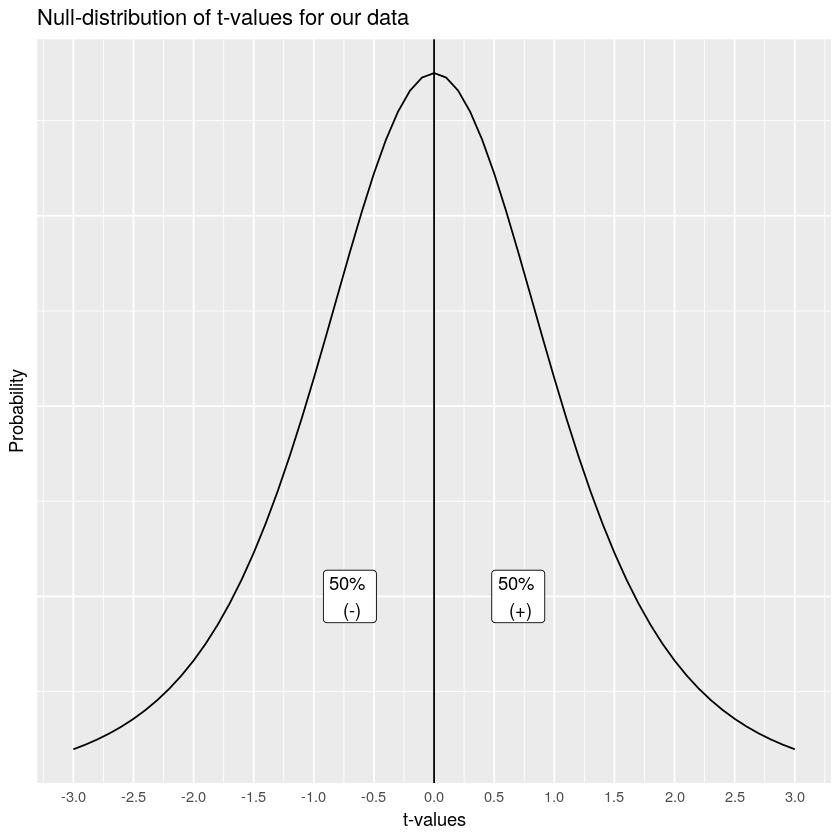Figure $$\PageIndex{1}$$: A distribution of t-values that can occur by chance alone, when there is no difference between the sample and a population.

The $$t$$-distribution above shows us the kinds of values $$t$$ will will take by chance alone, when we measure the mean differences for pairs of 5 samples (like our current). $$t$$ is most likely to be zero, which is good, because we are looking at the distribution of no-differences, which should most often be 0! But, sometimes, due to sampling error, we can get $$t$$s that are bigger than 0, either in the positive or negative direction. Notice the distribution is symmetrical, a $$t$$ from the null-distribution will be positive half of the time, and negative half of the time, that is what we would expect by chance.

So, what kind of information do we want know when we find a particular $$t$$ value from our sample? We want to know how likely the $$t$$ value like the one we found occurs just by chance. This is actually a subtly nuanced kind of question. For example, any particular $$t$$ value doesn’t have a specific probability of occurring. When we talk about probabilities, we are talking about ranges of probabilities. Let’s consider some probabilities. We will use the letter $$p$$, to talk about the probabilities of particular $$t$$ values.

1. What is the probability that $$t$$ is zero or positive or negative? The answer is p=1, or 100%. We will always have a $$t$$ value that is zero or non-zero…Actually, if we can’t compute the t-value, for example when the standard deviation is undefined, I guess then we would have a non-number. But, assuming we can calculate $$t$$, then it will always be 0 or positive or negative.
2. What is the probability of $$t$$ = 0 or greater than 0? The answer is p=.5, or 50%. 50% of $$t$$-values are 0 or greater.
3. What is the of $$t$$ = 0 or smaller than 0? The answer is p=.5, or 50%. 50% of $$t$$-values are 0 or smaller.

We can answer all of those questions just by looking at our t-distribution, and dividing it into two equal regions, the left side (containing 50% of the $$t$$ values), and the right side containing 50% of the $$t$$-values).

What if we wanted to take a more fine-grained approach, let’s say we were interested in regions of 10%. What kinds of $$t$$s occur 10% of the time. We would apply lines like the following. Notice, the likelihood of bigger numbers (positive or negative) gets smaller, so we have to increase the width of the bars for each of the intervals between the bars to contain 10% of the $$t$$-values, it looks like this: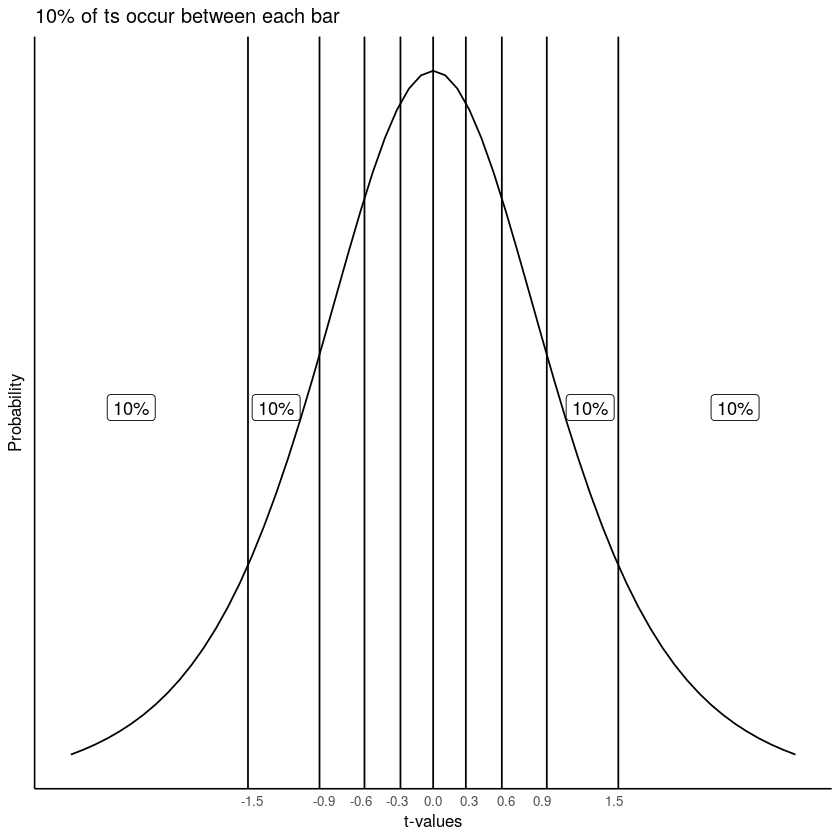Figure $$\PageIndex{2}$$: Splitting the t distribution up into regions each containing 5% of the t-values. The width between the bars narrows as they approach the center of the distribution, where there are more t-values.

Consider the probabilities ($$p$$) of $$t$$ for the different ranges.

1. $$t$$ <= -1.5 ($$t$$ is less than or equal to -1.5), $$p$$ = 10%
2. -1.5 >= $$t$$ <= -0.9 ($$t$$ is equal to or between -1.5 and -.9), $$p$$ = 10%
3. -.9 >= $$t$$ <= -0.6 ($$t$$ is equal to or between -.9 and -.6), $$p$$ = 10%
4. $$t$$ >= 1.5 ($$t$$ is greater than or equal to 1.5), $$p$$ = 10%

Notice, that the $$p$$s are always 10%. $$t$$s occur in these ranges with 10% probability.

## Getting the p-values for t-values

You might be wondering where I am getting some of these values from. For example, how do I know that 10% of $$t$$ values (for this null distribution) have a value of approximately 1.5 or greater than 1.5? The answer is I used R to tell me.

In most statistics textbooks the answer would be: there is a table at the back of the book where you can look these things up…This textbook has no such table. We could make one for you. And, we might do that. But, we didn’t do that yet…

So, where do these values come from, how can you figure out what they are? The complicated answer is that we are not going to explain the math behind finding these values because, 1) the authors (some of us) admittedly don’t know the math well enough to explain it, and 2) it would sidetrack us to much, 3) you will learn how to get these numbers in the lab with software, 4) you will learn how to get these numbers in lab without the math, just by doing a simulation, and 5) you can do it in R, or excel, or you can use an online calculator.

This is all to say that you can find the $$t$$s and their associated $$p$$s using software. But, the software won’t tell you what these values mean. That’s we are doing here. You will also see that software wants to know a few more things from you, such as the degrees of freedom for the test, and whether the test is one-tailed or two tailed. We haven’t explained any of these things yet. That’s what we are going to do now. Note, we explain degrees of freedom last. First, we start with a one-tailed test.

## One-tailed tests

A one-tailed test is sometimes also called a directional test. It is called a directional test, because a researcher might have a hypothesis in mind suggesting that the difference they observe in their means is going to have a particular direction, either a positive difference, or a negative difference.

Typically, a researcher would set an alpha criterion. The alpha criterion describes a line in the sand for the researcher. Often, the alpha criterion is set at p=.05. What does this mean? Let’s look at again at the graph of the $$t$$-distribution, and show the alpha criterion.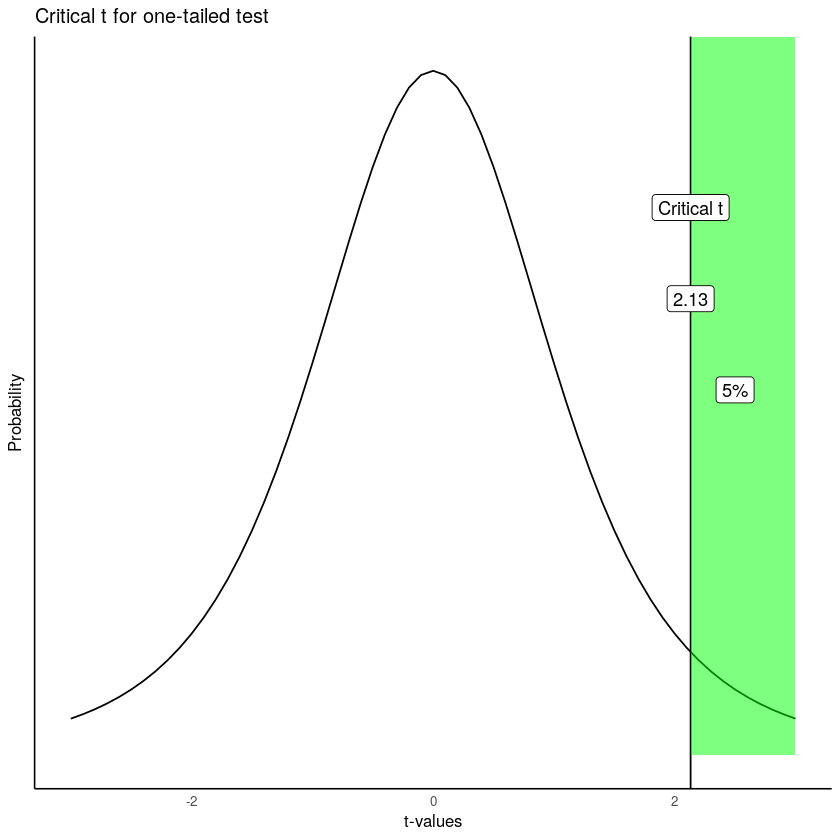Figure $$\PageIndex{3}$$: The critical value of t for an alpha criterion of 0.05. 5% of all ts are at this value or larger.

The figure shows that $$t$$ values of +2.13 or greater occur 5% of the time. Because the t-distribution is symmetrical, we also know that $$t$$ values of -2.13 or smaller also occur 5% of the time. Both of these properties are true under the null distribution of no differences. This means, that when there really are no differences, a researcher can expect to find $$t$$ values of 2.13 or larger 5% of the time.

Let’s review and connect some of the terms:

1. alpha criterion: the criterion set by the researcher to make decisions about whether they believe chance did or did not cause the difference. The alpha criterion here is set to p=.05
2. Critical $$t$$. The critical $$t$$ is the $$t$$-value associated with the alpha-criterion. In this case for a one-tailed test, it is the $$t$$ value where 5% of all $$t$$s are this number or greater. In our example, the critical $$t$$ is 2.13. 5% of all $$t$$ values (with degrees of freedom = 4) are +2.13, or greater than +2.13.
3. Observed $$t$$. The observed $$t$$ is the one that you calculated from your sample. In our example about the infants, the observed $$t$$ was $$t$$ (4) = 0.72.
4. p-value. The $$p$$-value is the probability of obtaining the observed $$t$$ value or larger. Now, you could look back at our previous example, and find that the $$p$$-value for $$t$$ (4) = .72, was p=.509. HOWEVER, this p-value was not calculated for a one-directional test…(we talk about what .509 means in the next section).

Let’s see what the $$p$$-value for $$t$$ (4) = .72 using a one-directional test would be, and what it would look like: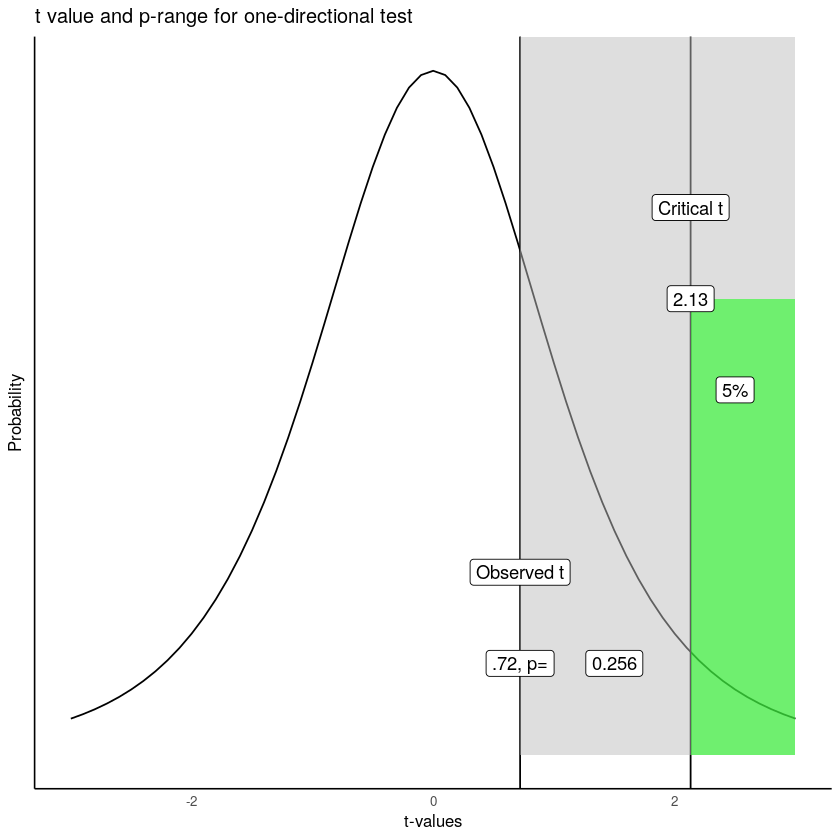Figure $$\PageIndex{4}$$: Critical value for a one-directional t-test.

Let’s take this one step at a time. We have located the observed $$t$$ of .72 on the graph. We shaded the right region all grey. What we see is that the grey region represents .256 or 25.6% of all $$t$$ values. In other words, 25.6% of $$t$$ values are 0.72 or larger than 0.72. You could expect, by chance alone, to a find a $$t$$ value of .72 or larger, 25.6% of the time. That’s fairly often. We did find a $$t$$ value of 0.72. Now that you know this kind of $$t$$ value or larger occurs 25.6% of the time, would you be confident that the mean difference was not due to chance? Probably not, given that chance can produce this difference fairly often.

Following the “standard” decision making procedure, we would claim that our $$t$$ value was not statistically significant, because it was not large enough. If our observed value was larger than the critical $$t$$ (larger than 2.13), defined by our alpha criterion, then we would claim that our $$t$$ value was statistically signicant. This would be equivalent to saying that we believe it is unlikely that the difference we observed was due to chance. In general, for any observed $$t$$ value, the associated $$p$$-value tells you how likely a $$t$$ of the observed size or larger would be observed. The $$p$$-value always refers to a range of $$t$$-values, never to a single $$t$$-value. Researchers use the alpha criterion of .05, as a matter of convenience and convention. There are other ways to interpret these values that do not rely on a strict (significant versus not) dichotomy.

## Two-tailed tests

OK, so that was one-tailed tests… What are two tailed tests, what is that? The $$p$$-value that we originally calculated from our paired-samples $$t$$-test was for a 2-tailed test. Often, the default is that the $$p$$-value is for a two-tailed test.

The two-tailed test, is asking a more general question about whether a difference is likely to have been produced by chance. The question is: what is probability of any difference. It is also called a non-directional test, because here we don’t care about the direction or sign of the difference (positive or negative), we just care if there is any kind of difference.

The same basic things as before are involved. We define an alpha criterion ($$\alpha = 0.05$$). And, we say that any observed $$t$$ value that has a probability of $$p$$ <.05 ($$p$$ is less than .05) will be called statistically signficant, and ones that are more likely ($$p$$ >.05, $$p$$ is greater than .05) will be called null-results, or not statistically significant. The only difference is how we draw the alpha range. Before it was on the right side of the $$t$$ distribution (we were conducting a one-sided test remember, so we were only interested in one side).

Let’s just take a look at what the most extreme 5% of the t-values are, when we ignore if they are positive or negative: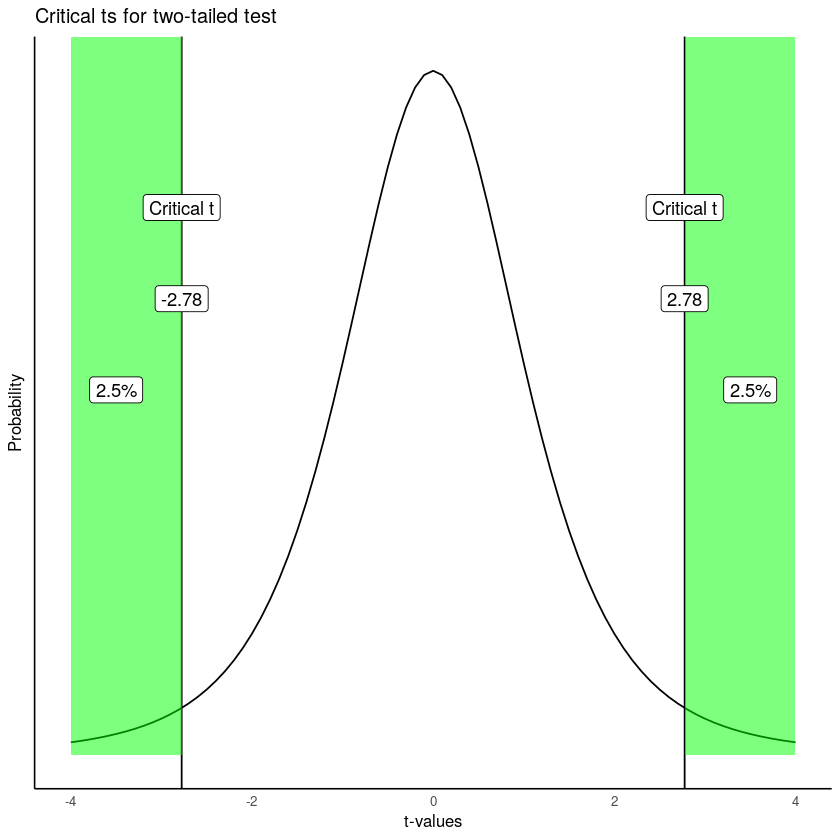Figure $$\PageIndex{5}$$: Critical values for a two-tailed test. Each line represents the location where 2.5% of all ts are larger or smaller than critical value. The total for both tails is 5%.

Here is what we are seeing. A distribution of no differences (the null, which is what we are looking at), will produce $$t$$s that are 2.78 or greater 2.5% of the time, and $$t$$s that are -2.78 or smaller 2.5% of the time. 2.5% + 2.5% is a total of 5% of the time. We could also say that $$t$$s larger than +/- 2.78 occur 5% of the time.

As a result, the critical $$t$$ value is (+/-) 2.78 for a two-tailed test. As you can see, the two-tailed test is blind to the direction or sign of the difference. Because of this, the critical $$t$$ value is also higher for a two-tailed test, than for the one-tailed test that we did earlier. Hopefully, now you can see why it is called a two-tailed test. There are two tails of the distribution, one on the left and right, both shaded in green.

## One or two tailed, which one?

Now that you know there are two kinds of tests, one-tailed, and two-tailed, which one should you use? There is some conventional wisdom on this, but also some debate. In the end, it is up to you to be able to justify your choice and why it is appropriate for you data. That is the real answer.

The conventional answer is that you use a one-tailed test when you have a theory or hypothesis that is making a directional prediction (the theory predicts that the difference will be positive, or negative). Similarly, use a two-tailed test when you are looking for any difference, and you don’t have a theory that makes a directional prediction (it just makes the prediction that there will be a difference, either positive or negative).

Also, people appear to choose one or two-tailed tests based on how risky they are as researchers. If you always ran one-tailed tests, your critical $$t$$ values for your set alpha criterion would always be smaller than the critical $$t$$s for a two-tailed test. Over the long run, you would make more type I errors, because the criterion to detect an effect is a lower bar for one than two tailed tests.

Remember type 1 errors occur when you reject the idea that chance could have caused your difference. You often never know when you make this error. It happens anytime that sampling error was the actual cause of the difference, but a researcher dismisses that possibility and concludes that their manipulation caused the difference.

Similarly, if you always ran two-tailed tests, even when you had a directional prediction, you would make fewer type I errors over the long run, because the $$t$$ for a two-tailed test is higher than the $$t$$ for a one-tailed test. It seems quite common for researchers to use a more conservative two-tailed test, even when they are making a directional prediction based on theory. In practice, researchers tend to adopt a standard for reporting that is common in their field. Whether or not the practice is justifiable can sometimes be an open question. The important task for any researcher, or student learning statistics, is to be able to justify their choice of test.

## Degrees of freedom

Before we finish up with paired-samples $$t$$-tests, we should talk about degrees of freedom. Our sense is that students don’t really understand degrees of freedom very well. If you are reading this textbook, you are probably still wondering what is degrees of freedom, seeing as we haven’t really talked about it all.

For the $$t$$-test, there is a formula for degrees of freedom. For the one-sample and paired sample $$t$$-tests, the formula is:

$$\text{Degrees of Freedom} = \text{df} = n-1$$. Where n is the number of samples in the test.

In our paired $$t$$-test example, there were 5 infants. Therefore, degrees of freedom = 5-1 = 4.

OK, that’s a formula. Who cares about degrees of freedom, what does the number mean? And why do we report it when we report a $$t$$-test… you’ve probably noticed the number in parentheses e.g., $$t$$(4)=.72, the 4 is the $$df$$, or degrees of freedom.

Degrees of freedom is both a concept, and a correction. The concept is that if you estimate a property of the numbers, and you use this estimate, you will be forcing some constraints on your numbers.

Consider the numbers: 1, 2, 3. The mean of these numbers is 2. Now, let’s say I told you that the mean of three numbers is 2. Then, how many of these three numbers have freedom? Funny question right. What we mean is, how many of the three numbers could be any number, or have the freedom to be any number.

The first two numbers could be any number. But, once those two numbers are set, the final number (the third number), MUST be a particular number that makes the mean 2. The first two numbers have freedom. The third number has no freedom.

To illustrate. Let’s freely pick two numbers: 51 and -3. I used my personal freedom to pick those two numbers. Now, if our three numbers are 51, -3, and x, and the mean of these three numbers is 2. There is only one solution, x has to be -42, otherwise the mean won’t be 2. This is one way to think about degrees of freedom. The degrees of freedom for these three numbers is n-1 = 3-1= 2, because 2 of the numbers can be free, but the last number has no freedom, it becomes fixed after the first two are decided.

Now, statisticians often apply degrees of freedom to their calculations, especially when a second calculation relies on an estimated value. For example, when we calculate the standard deviation of a sample, we first calculate the mean of the sample right! By estimating the mean, we are fixing an aspect of our sample, and so, our sample now has n-1 degrees of freedom when we calculate the standard deviation (remember for the sample standard deviation, we divide by n-1…there’s that n-1 again.)

### Simulating how degrees of freedom affects the t distribution

There are at least two ways to think the degrees of freedom for a $$t$$-test. For example, if you want to use math to compute aspects of the $$t$$ distribution, then you need the degrees of freedom to plug in to the formula… If you want to see the formulas I’m talking about, scroll down on the t-test Wikipedia page and look for the probability density or cumulative distribution functions…We think that is quite scary for most people, and one reason why degrees of freedom are not well-understood.

If we wanted to simulate the $$t$$ distribution we could more easily see what influence degrees of freedom has on the shape of the distribution. Remember, $$t$$ is a sample statistic, it is something we measure from the sample. So, we could simulate the process of measuring $$t$$ from many different samples, then plot the histogram of $$t$$ to show us the simulated $$t$$ distribution.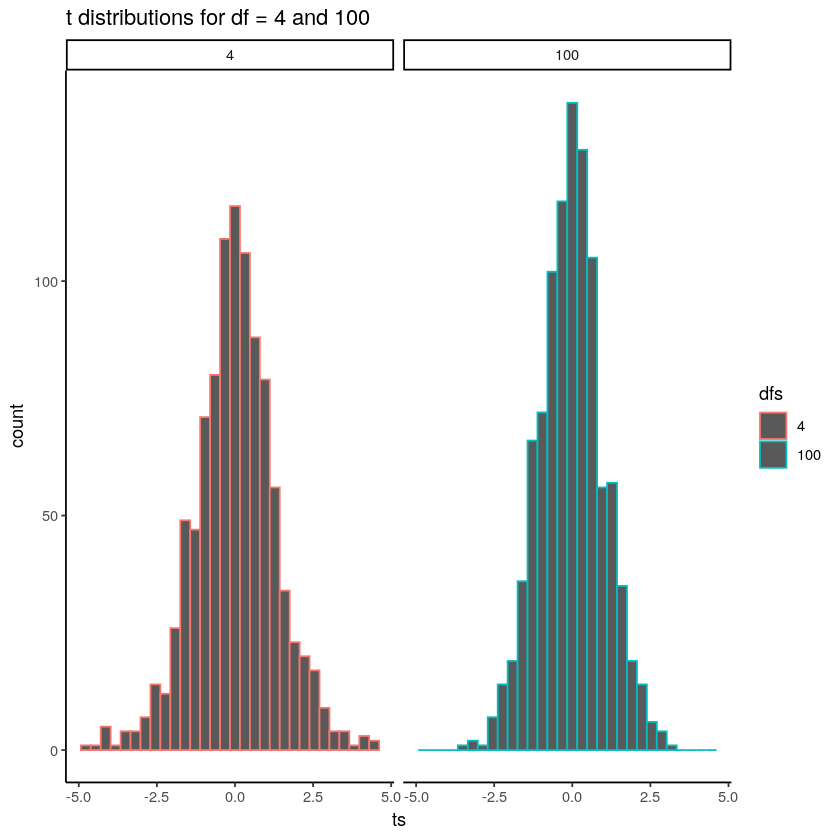Figure $$\PageIndex{6}$$: The width of the t distribution shrinks as sample size increases.

Notice that the red distribution for $$df$$ =4, is a little bit shorter, and a little bit wider than the bluey-green distribution for $$df$$ = 100. As degrees of freedom increase, the $$t$$-distribution gets taller (in the middle), and narrower in the range. It get’s more peaky. Can you guess the reason for this? Remember, we are estimating a sample statistic, and degrees of freedom is really just a number that refers to the number of subjects (well minus one). And, we already know that as we increase $$n$$, our sample statistics become better estimates (less variance) of the distributional parameters they are estimating. So, $$t$$ becomes a better estimate of it’s “true” value as sample size increase, resulting in a more narrow distribution of $$t$$s.

There is a slightly different $$t$$ distribution for every degrees of freedom, and the critical regions associated with 5% of the extreme values are thus slightly different every time. This is why we report the degrees of freedom for each t-test, they define the distribution of $$t$$ values for the sample-size in question. Why do we use n-1 and not n? Well, we calculate $$t$$ using the sample standard deviation to estimate the standard error or the mean, that estimate uses n-1 in the denominator, so our $$t$$ distribution is built assuming n-1. That’s enough for degrees of freedom…

This page titled 6.3: Paired-samples t-test is shared under a CC BY-SA 4.0 license and was authored, remixed, and/or curated by Matthew J. C. Crump via source content that was edited to the style and standards of the LibreTexts platform; a detailed edit history is available upon request.